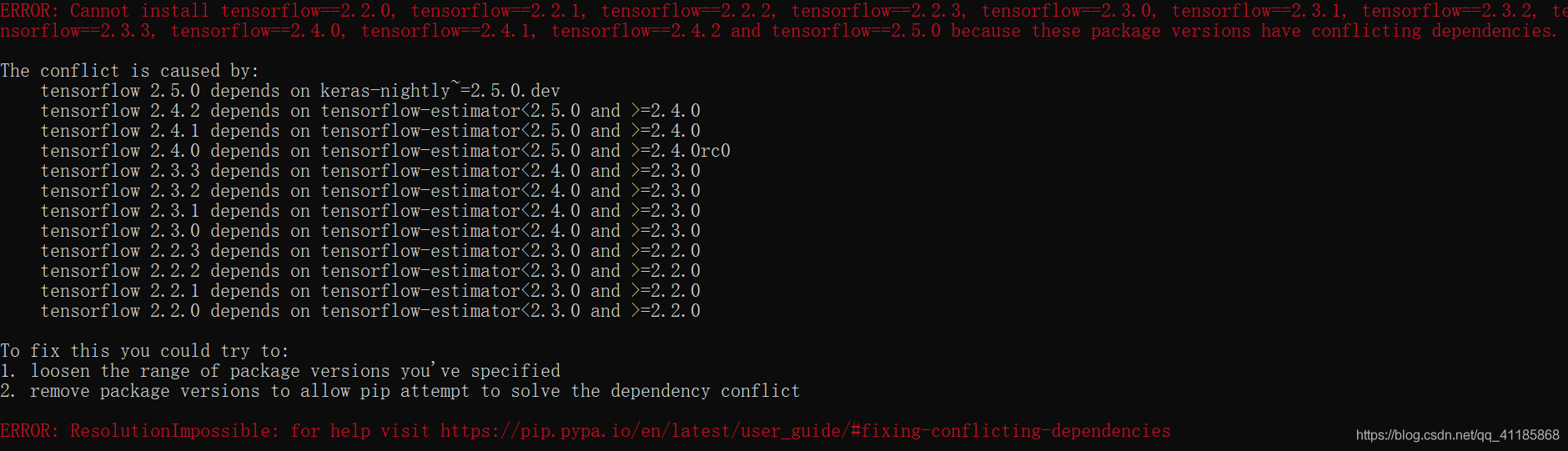# 成功解决To fix this you could try to: 1. loosen the range of package versions you‘ve specified ​​​​​​​

+关注继续查看

## 解决问题he conflict is caused by:

tensorflow 2.5.0 depends on keras—nightly=2.5.0.dev

tensorflow 2.4.2 depends on tensorflow—estimator<2.5.0 and >=2.4.0

tensorflow 2.4.1 depends on tensorflow—estimator<2.5.0 and >=2.4.0

tensorflow 2.4.0 depends on tensorflow—estimator<2.5.0 and >=2.4.0rc0

tensorflow 2.3.3 depends on tensorflow—estimator<2.4.0 and >=2.3.0

tensorflow 2.3.2 depends on tensorflow—estimator<2.4.0 and >=2.3.0

tensorflow 2.3.1 depends on tensorflow—estimator<2.4.0 and >=2.3.0

tensorflow 2.3.0 depends on tensorflow—estimator<2.4.0 and >=2.3.0

tensorflow 2.2.3 depends on tensorflow—estimator<2.3.0 and >=2.2.0

tensorflow 2.2.2 depends on tensorflow—estimator<2.3.0 and >=2.2.0

tensorflow 2.2.1 depends on tensorflow—estimator<2.3.0 and >=2.2.0

tensorflow 2.2.0 depends on tensorflow—estimator<2.3.0 and >=2.2.0

To fix this you could try to:

1. loosen the range of package versions you've specified

2. remove package versions to allow pip attempt to solve the dependency conflict

## 解决思路

1. 放宽您指定的包版本的范围

2. 删除包版本以允许PIP尝试解决依赖项冲突

## 解决方法9251 06777 0Xshell使用SSH远程登录阿里云ECS服务器CentOS7
10066 07718 0windows server 2008阿里云ECS服务器安全设置

5357 09394 02109 01997 016683 046713 0

1701

0

《SaaS模式云原生数据仓库应用场景实践》

《看见新力量：二》电子书